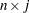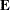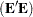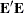# Overview: PRINCOMP Procedure

The PRINCOMP procedure performs principal component analysis. As input you can use raw data, a correlation matrix, a covariance matrix, or a sum-of-squares-and-crossproducts (SSCP) matrix. You can create output data sets containing eigenvalues, eigenvectors, and standardized or unstandardized principal component scores.

Principal component analysis is a multivariate technique for examining relationships among several quantitative variables. The choice between using factor analysis and using principal component analysis depends in part on your research objectives. You should use the PRINCOMP procedure if you are interested in summarizing data and detecting linear relationships. You can use principal components to reduce the number of variables in regression, clustering, and so on. See Chapter 9, Introduction to Multivariate Procedures, for a detailed comparison of the PRINCOMP and FACTOR procedures.

You can use ODS Graphics to display the scree plot, component pattern plot, component pattern profile plot, matrix plot of component scores, and component score plots. These plots are especially valuable tools in exploratory data analysis.

Principal component analysis was originated by Pearson (1901) and later developed by Hotelling (1933). The application of principal components is discussed by Rao (1964), Cooley and Lohnes (1971), and Gnanadesikan (1977). Excellent statistical treatments of principal components are found in Kshirsagar (1972), Morrison (1976), and Mardia, Kent, and Bibby (1979).

Given a data set with p numeric variables, you can compute p principal components. Each principal component is a linear combination of the original variables, with coefficients equal to the eigenvectors of the correlation or covariance matrix. The eigenvectors are customarily taken with unit length. The principal components are sorted by descending order of the eigenvalues, which are equal to the variances of the components.

Principal components have a variety of useful properties (Rao; 1964; Kshirsagar; 1972):

• The eigenvectors are orthogonal, so the principal components represent jointly perpendicular directions through the space of the original variables.

• The principal component scores are jointly uncorrelated. Note that this property is quite distinct from the previous one.

• The first principal component has the largest variance of any unit-length linear combination of the observed variables. The jth principal component has the largest variance of any unit-length linear combination orthogonal to the firstprincipal components. The last principal component has the smallest variance of any linear combination of the original variables.

• The scores on the first j principal components have the highest possible generalized variance of any set of unit-length linear combinations of the original variables.

• The first j principal components provide a least squares solution to the modelwhereis anmatrix of the centered observed variables;is thematrix of scores on the first j principal components;is thematrix of eigenvectors;is anmatrix of residuals; and you want to minimize trace, the sum of all the squared elements in. In other words, the first j principal components are the best linear predictors of the original variables among all possible sets of j variables, although any nonsingular linear transformation of the first j principal components would provide equally good prediction. The same result is obtained if you want to minimize the determinant or the Euclidean (Schur, Frobenious) norm ofrather than the trace.

• In geometric terms, the j-dimensional linear subspace spanned by the first j principal components provides the best possible fit to the data points as measured by the sum of squared perpendicular distances from each data point to the subspace. This is in contrast to the geometric interpretation of least squares regression, which minimizes the sum of squared vertical distances. For example, suppose you have two variables. Then, the first principal component minimizes the sum of squared perpendicular distances from the points to the first principal axis. This is in contrast to least squares, which would minimize the sum of squared vertical distances from the points to the fitted line.

Principal component analysis can also be used for exploring polynomial relationships and for multivariate outlier detection (Gnanadesikan; 1977), and it is related to factor analysis, correspondence analysis, allometry, and biased regression techniques (Mardia, Kent, and Bibby; 1979).